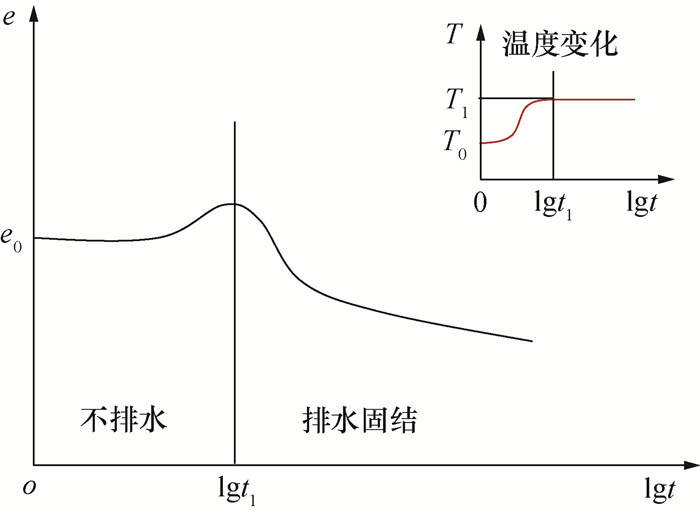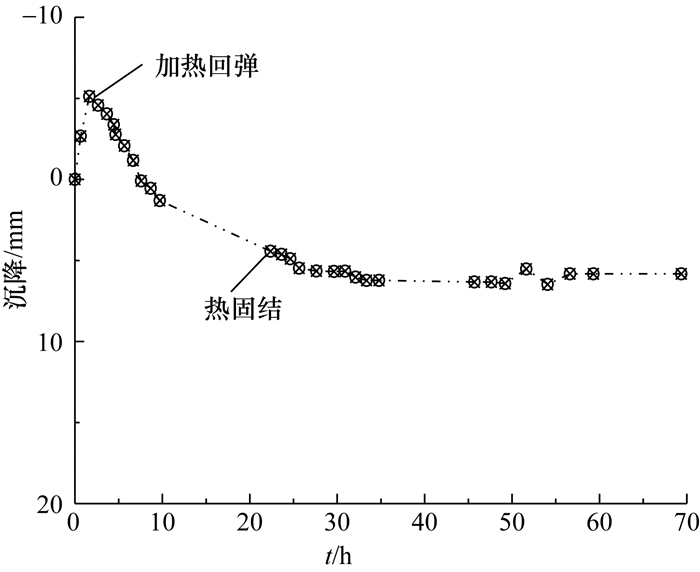, 毛伟赟1, 孔纲强2, 程冠初1
1. 宁波大学 岩土工程研究所, 宁波 315211;
2. 河海大学 岩土力学与堤坝工程教育部重点试验室, 南京 210024

Effective stress principle in saturated soil with the effect of temperature
, MAO Weiyun1, KONG Gangqiang2, CHENG Guanchu1
1. Institute of Geotechnical Engineering, Ningbo University, Ningbo 315211, China;
2. Key Laboratory of Geomechanics and Embankment Engineering of Ministry of Education, Hohai University, Nanjing 210024, China
Abstract: Thermo-mechanical coupling is a key research topic in geotechnical engineering today for solving thermal geotechnical engineering problems, including underground energy engineering projects, radioactive waste disposal, oil transport and thermal pipelines, and thermal soft ground stabilization methods. The classical principle of the effective stress in soil mechanics describes the pressure distribution between the soil skeleton and the pore fluid for saturated soil. However, the pressure distribution and its changes between the soil skeleton and the pore water with thermal-mechanical coupling have rarely been reported. This study combines the macroscopic thermal response of the soil consolidation with the classical effective stress principle to analyze the influence of heating on the soil skeleton and the pore water to develop an effective stress and pore pressure prediction formula that includes the influence of temperature. The effective stress principle considering the temperature effect is used to analyze the effective stress and pore pressure distributions for various displacement and drainage boundary conditions. The results show that the thermo-mechanical coupling affects the total stress, the excess pore water pressure and the effective stress in the soil. The temperature stress and the thermal excess pore pressure caused by heating change with time and affect the soil consolidation and compression. The thermal stresses affect the soil stress state and indirectly affect the soil compressibility and strength. The research results can be used to develop thermal consolidation theory and to analyze problems related to thermal geotechnical engineering.
Key words: effective stress principle    temperature    effective stress    pore water pressure    consolidation

1 软土和软土地基的热固结响应图 1 土单元热固结试验结果图 2 软土地基热固结试验结果

2 考虑温度影响的有效应力原理图 3 有效应力原理分析

2.1 加热引起的土骨架应力

 ${\varepsilon _{\rm{T}}} = {\alpha _{\rm{s}}}\Delta T.$ (1)

 $\sigma _{\rm{T}}^\prime = {\alpha _{\rm{s}}}\Delta T{E_{\rm{r}}}.$ (2)

 $\sigma _{{\rm{qT}}}^\prime = \sigma _{\rm{q}}^\prime + \sigma _{\rm{T}}^\prime .$ (3)

2.2 加热引起的孔隙水压力

 ${u_{{\rm{T0}}}} = \frac{{[{n_{\rm{v}}}({\alpha _{\rm{f}}} - {\alpha _{\rm{s}}}) + {\alpha _{{\rm{st}}}}]\Delta T}}{{{m_{\rm{v}}} + {n_{\rm{v}}}{m_{\rm{w}}}}}.$ (4)

 ${u_{{\rm{T0}}}} = \frac{{[{n_{\rm{v}}}({\alpha _{\rm{f}}} - {\alpha _{\rm{s}}}) + {\alpha _{{\rm{st}}}}]\Delta T}}{{{m_{\rm{v}}}}}.$ (5)

 ${\alpha _{\rm{f}}} = (139 + 6.1T) \times {10^{ - 6}}.$ (6)

 $\begin{array}{*{20}{l}} {{\alpha _{\rm{f}}} = {\alpha _0} + ({\alpha _1} + {\beta _1}T){\rm{ln}}({m_{\rm{T}}}p) + }\\ {{\kern 1pt} {\kern 1pt} {\kern 1pt} {\kern 1pt} {\kern 1pt} {\kern 1pt} {\kern 1pt} {\kern 1pt} {\kern 1pt} {\kern 1pt} {\kern 1pt} {\kern 1pt} {\kern 1pt} {\kern 1pt} {\kern 1pt} {\kern 1pt} {\kern 1pt} {\kern 1pt} {\kern 1pt} {\kern 1pt} {\kern 1pt} ({\alpha _2} + {\beta _2}T){{({\rm{ln}}({m_{\rm{T}}}p))}^2}.} \end{array}$ (7)

 ${u_{{\rm{qT0}}}} = {u_{{\rm{q0}}}} + {u_{{\rm{T0}}}}.$ (8)

 ${u_{{\rm{q0}}}} = {q_0}.$ (9)

 ${u_{{\rm{qT}}}} = {u_{\rm{q}}} + {u_{\rm{T}}}.$ (10)
2.3 加热引起的约束力

 ${\sigma _{{\rm{qT}}}} = {\sigma _{\rm{q}}} + {\sigma _{{\rm{RT}}}}.$ (11)

 ${{\sigma _{{\rm{qT}}}} = \sigma _{{\rm{qT}}}^\prime + {u_{{\rm{qT}}}}.}$ (12)

 ${{\sigma _{\rm{q}}} + {\sigma _{{\rm{RT}}}} = \sigma _{\rm{q}}^\prime + \sigma _{\rm{T}}^\prime + {u_{\rm{q}}} + {u_{\rm{T}}}.}$ (13)

 $\sigma _{\rm{q}}^\prime = {\rm{max}}\{ 0,{\sigma _{\rm{q}}} - {u_{{\rm{qT}}}}\} .$ (14)

 固结时间 约束不排水 约束排水 自由不排水 自由排水 t=0 uq=q0uT=uT0σ′q=0σ′T=σ′T0 u=q0uT=uT0σ′q=0σ′T=σ′T0 uq=q0uT=uT0σ′q=0σ′T=σ′T0 uq=q0uT=uT0σ′q=0σ′T=σ′T0 t>0 uq=q0uT=uT0σ′q=0σ′T=σ′T0 uq减小uT减小σ′q增大σ′T=σ′T0 uq=q0uT减小σ′q=0σ′T=0 uq减小uT减小σ′q增大σ′T=0 t→∞ uq=q0uT=uT0σ′q=0σ′T=σ′T0 uq=q0uT=0σ′q=q0σ′T=σ′T0 uq=q0uT < uT0σ′q=0σ′T=0 uq=q0uT=0σ′q=0σ′T=0 注：q0为瞬时施加且恒定的外荷载。

3 影响因素与讨论 3.1 加热对结合水的影响

3.2 热膨胀系数

3.3 热应力和约束力的时间效应

3.4 变温情况

 ${\beta _t} = \left\{ {\begin{array}{*{20}{l}} {\frac{{{T_t} - {T_0}}}{{{T_{\rm{f}}} - {T_0}}},}&{0 \le t < {t_1};}\\ {1,}&{t \ge {t_1}.} \end{array}} \right.$ (15)

 ${u_{{\rm{qT}}}} = {u_{\rm{q}}} + {\beta _t}{u_{\rm{T}}}.$ (16)

t1=0，则βt=1，式(16)退化为式(10)，即加热瞬时完成情况。

3.5 有效应力原理的应用

4 计算与分析 4.1 加热产生的膨胀应力

 ΔT/℃ αs/(℃-1) Er/MPa σ′T/kPa 30 1.0×10-6 5 0.15 60 1.0×10-5 10 6.00

4.2 加热产生的初始热孔压

 p/MPa T/℃ ΔT/℃ αf/(10-4℃-1) (αs=αst)/(10-5℃-1) nv mv/(MPa-1) uT0/kPa 0.1 40 20 4.7 1.0 0.5 0.33 15 0.2 80 60 4.5 1.0 0.5 0.33 27 0.2 80 60 4.5 1.0 0.5 0.20 46

4.3 应力分担随时间变化

 参数 取值 Er/MPa 5.00 Cv/(cm2·s-2) 1.0×10-4 nv 0.50 mv/(MPa-1) 0.33 αs/(℃-1) 1.0×10-5 αst/(℃-1) 1.0×10-5 T0/℃ 20 ΔT/℃ 60图 4 应力分担随时间变化

5 结语

1) 相比于常温下的有效应力原理，热-力耦合作用下土体外围应力、有效应力和孔隙水压力之间的关系不变。但是，各应力的内涵及其分担比例与常温下的有效应力原理不同。

2) 有效应力包括荷载和温度变化引起的土骨架应力。土骨架上的热应力随时间变化而变化；在常见加热温度变化范围内(变化量在60 ℃以内)，土骨架上的热应力较小，对饱和土体的固结沉降影响不大。

3) 孔隙水压力包括荷载和温度变化引起的孔隙水压力。加热使饱和土中产生较为明显的热孔压；在有排水条件下，热孔压随时间逐渐消散，并影响土体的排水固结过程和土体压缩量；热孔压改变土颗粒间的接触力，进而间接影响土体的压缩特性和剪切强度。

4) 土体的热膨胀趋势使得土体受到约束力的作用。约束力的大小与土骨架上的膨胀应力和热孔压有关。对于常见的涉热岩土工程问题，可以忽略饱和土土骨架上膨胀应力和外围约束力的时间效应。

  李广信. 有效应力原理能够推翻吗[J]. 岩土工程界, 2007, 10(7): 22-26. LI G X. Can the principle of effective stress be overthrew[J]. Geotechnical Engineering World, 2007, 10(7): 22-26. DOI:10.3969/j.issn.1674-7801.2007.07.006 (in Chinese)  陈津民. 三谈饱和土的有效应力:和李广信老师商榷[J]. 岩土工程界, 2009, 12(11): 1-3. CHEN J M. A third discussion on effective stress principle of saturated soil with LI Guangxin[J]. Geotechnical Engineering World, 2009, 12(11): 1-3. DOI:10.3969/j.issn.1674-7801.2009.11.002 (in Chinese)  李广信. 关于有效应力原理的几个问题[J]. 岩土工程学报, 2011, 33(2): 315-320. LI G X. Some problems about principle of effective stress[J]. Chinese Journal of Geotechnical Engineering, 2011, 33(2): 315-320. (in Chinese)  邵龙潭. 饱和土的土骨架应力方程[J]. 岩土工程学报, 2011, 33(12): 1833-1837. SHAO L T. Skeleton stress equation for saturated soils[J]. Chinese Journal of Geotechnical Engineering, 2011, 33(12): 1833-1837. (in Chinese)  路德春, 杜修力, 许成顺. 有效应力原理解析[J]. 岩土工程学报, 2013, 35(S1): 146-151. LU D C, DU X L, XU C S. Analytical solutions to principle of effective stress[J]. Chinese Journal of Geotechnical Engineering, 2013, 35(S1): 146-151. (in Chinese)  杜修力, 张佩, 许成顺, 等. 论有效应力原理与有效应力[J]. 岩土工程学报, 2018, 40(3): 486-494. DU X L, ZHANG P, XU C S, et al. On principle of effective stress and effective stress[J]. Chinese Journal of Geotechnical Engineering, 2018, 40(3): 486-494. (in Chinese)  李广信, 张丙印, 于玉贞. 土力学[M]. 2版. 北京: 清华大学出版社, 2013. LI G X, ZHANG B Y, YU Y Z. Soil mechanics[M]. 2nd ed. Beijing: Tsinghua University Press, 2013. (in Chinese)  陶海冰.热流固作用下软土静动力学特性及应用[D].杭州: 浙江大学, 2015. TAO H B. The thermo-hydro-mechanical effect on static and dynamic properties of soft soil and its application[D]. Hangzhou: Zhejiang University, 2015. (in Chinese)  王宽君.软土性状的温度效应[D].杭州: 浙江大学, 2017. WANG K J. Temperature dependent behavior of soft soils[D]. Hangzhou: Zhejiang University, 2017. (in Chinese)  CAMPANELLA R G, MITCHELL J K. Influence of temperature variations on soil behavior[J]. ASCE Journal of the Soil Mechanics and Foundations Division, 1968, 94(SM3): 709-734.  程晓辉, 陈志辉. 纯主应力旋转条件下饱和黏土累积变形的热力学模型分析[J]. 岩土工程学报, 2015, 37(9): 1581-1590. CHENG X H, CHEN Z H. Thermodynamic modeling of accumulated deformation of saturated clays under pure principal stress rotation[J]. Chinese Journal of Geotechnical Engineering, 2015, 37(9): 1581-1590. (in Chinese)  陈卫忠, 龚哲, 于洪丹, 等. 黏土岩温度-渗流-应力耦合特性试验与本构模型研究进展[J]. 岩土力学, 2015, 36(5): 1217-1238. CHEN W Z, GONG Z, YU H D, et al. Review of thermo-hydro-mechanical coupled tests and constitutive models of clays[J]. Rock and Soil Mechanics, 2015, 36(5): 1217-1238. (in Chinese)  LIU Q, DENG Y B, WANG T Y. One-dimensional nonlinear consolidation theory for soft ground considering secondary consolidation and the thermal effect[J]. Computers and Geotechnics, 2018, 104: 22-28. DOI:10.1016/j.compgeo.2018.08.007  LALOUI L, DI DONNA A.能源地下结构[M].孔纲强, 译.北京: 中国建筑工业出版社, 2016. LALOUI L, DI DONNA A. Energy geostructures: Innovation in underground engineering[M]. KONG G Q, trans. Beijing: China Architecture and Building Press, 2016. (in Chinese)  中华人民共和国住房和城乡建设部.桩基地热能利用技术标准: JGJ/T438-2018[S].北京: 中国建筑工业出版社, 2018. Ministry of Housing and Urban-Rural Development of the People's Republic of China. Technical standard for pile geothermal energy utilization: JGJ/T438-2018[S]. Beijing: China Architecture and Building Press, 2018. (in Chinese)  ABUEL-NAGA H M, BERGADO D T, CHAIPRAKAIKEOW S. Innovative thermal technique for enhancing the performance of prefabricated vertical drain during the preloading process[J]. Geotextiles and Geomembranes, 2006, 24(6): 359-370. DOI:10.1016/j.geotexmem.2006.04.003  BALDI G, HUECKEL T, PELLEGRINI R. Thermal volume changes of the mineral-water system in low-porosity clay soils[J]. Canadian Geotechnical Journal, 1988, 25(4): 807-825. DOI:10.1139/t88-089  CUI Y J, SULTAN N, DELAGE P. A thermomechanical model for saturated clays[J]. Canadian Geotechnical Journal, 2000, 37(3): 607-620. DOI:10.1139/t99-111  FRANÇOIS B, LALOUI L. ACMEG-TS:A constitutive model for unsaturated soils under non-isothermal conditions[J]. International Journal for Numerical and Analytical Methods in Geomechanics, 2008, 32(16): 1955-1988. DOI:10.1002/nag.712  ABUEL-NAGA H M, BERGADO D T, BOUAZZA A, et al. Volume change behaviour of saturated clays under drained heating conditions:Experimental results and constitutive modeling[J]. Canadian Geotechnical Journal, 2007, 44(8): 942-956. DOI:10.1139/t07-031  HONG P Y, PEREIRA J M, TANG A M, et al. On some advanced thermo-mechanical models for saturated clays[J]. International Journal for Numerical and Analytical Methods in Geomechanics, 2013, 37(17): 2952-2971. DOI:10.1002/nag.2170  COCCIA C J R, MCCARTNEY J S. Thermal volume change of poorly draining soils II:Model development and experimental validation[J]. Computers and Geotechnics, 2016, 80: 16-25. DOI:10.1016/j.compgeo.2016.06.010  邓岳保, 王天园, 孔纲强. 考虑温度效应的饱和土地基固结理论[J]. 岩土工程学报, 2019, 41(10): 1827-1835. DENG Y B, WANG T Y, KONG G Q. Consolidation theory for saturated ground considering temperature effect[J]. Chinese Journal of Geotechnical Engineering, 2019, 41(10): 1827-1835. (in Chinese)